StatLect

# Zero-probability events

This lecture defines zero-probability events and discusses some counterintuitive aspects of their apparently simple definition, in particular the fact that a zero-probability event is not an event that never happens.

There are common probabilistic settings in which zero-probability events do happen all the time!## Definition

As we said, the definition is very simple.

Definition Letbe an event and denote its probability by. We say thatis a zero-probability event if and only ifDespite the simplicity of the definition, there are some features of zero-probability events that might seem paradoxical.

## Example

We illustrate these features with the following example.

### A continuous sample space

Consider a probabilistic experiment whose set of possible outcomes, called sample space and denoted by, is the unit interval:It is possible to assign probabilities in such a way that each sub-interval has probability equal to its length:The proof that such an assignment of probabilities can be consistently performed is beyond the scope of this example, but you can find it in any elementary measure theory book (e.g. Williams - 1991).

### All the possible outcomes have zero probability

As a direct consequence of this assignment, all the possible outcomeshave zero probability: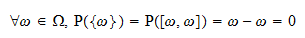Stated differently, every possible outcome is a zero-probability event.

This might seem counterintuitive. In everyday language, a zero-probability event is an event that never happens.

However, this example illustrates that a zero-probability event can indeed happen.

Since the sample space provides an exhaustive description of the possible outcomes, one and only one of the sample pointswill be the realized outcome.

But we have just demonstrated that all the sample points are zero-probability events: as a consequence, the realized outcome can only be a zero-probability event.

### Another counter-intuitive property

Another apparently paradoxical aspect of this probability model is that the sample spacecan be obtained as the union of disjoint zero-probability events:where eachis a zero-probability event and all events in the union are disjoint.

If we forgot that the additivity property of probability applies only to countable collections of subsets, we would mistakenly deduce that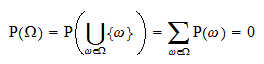and we would come to a contradiction:, when, by the properties of probability, it should be.

Of course, the fallacy in such an argument is thatis not a countable set and, hence, the additivity property cannot be used.

### Main take-away

The main lesson to be taken from this example is that a zero-probability event is not an event that never happens (also called an impossible event): in some probability models, where the sample space is not countable, zero-probability events do happen all the time!

## Understanding the example

The reason for the apparent paradoxes is that the sample space described above is uncountable. It has the power of the continuum.

Sample spaces of this kind are very common in statistics. They implicitly arise every time that we define a continuous random variable.

But why do we define mathematical objects that have such counterintuitive properties?

If you are eager to read an answer to this question and you know what a random variable is, you can read our page on continuous random variables.

## Almost sure and almost surely

The notion of a zero-probability event plays a special role in probability theory and statistics because it underpins the important concepts of almost sure property and almost sure event.

Often, we want to prove that some property is almost always satisfied, or something happens almost always.

"Almost always" means that the property is satisfied for all sample points, except possibly for a negligible set of sample points.

The concept of zero-probability event is used to determine which sets are negligible: if a set is included in a zero-probability event, then it is negligible.

Definition Letbe some property that a sample pointcan either satisfy or not satisfy. Letbe the set of all sample points that satisfy the property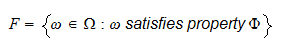Denote its complement (the set of all points not satisfying property) by. Propertyis said to be almost sure if there exists a zero-probability eventsuch that.

An almost sure property is said to hold almost surely (often abbreviated as a.s.). Sometimes, an almost sure property is also said to hold with probability one (abbreviated w.p.1).

## Almost sure events

Remember (see the lecture on probability) that some subsets of the sample space may not be considered events.

The above definition of almost sure property allows us to consider also setsthat are not, strictly speaking, events.

However, in the case in whichis an event,is called an almost sure event and we say thathappens almost surely.

Furthermore, since there exists an eventsuch thatand, we can apply the monotonicity of probability:which in turn implies. Finally, recalling the formula for the probability of a complement:Thus, an almost sure event is an event that happens with probability.

## Example of almost sure event

Consider the sample spaceand the assignment of probabilities introduced in the previous example:We want to prove that the event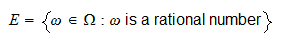is a zero-probability event.

Since the set of rational numbers is countable andis a subset of the set of rational numbers,is countable.

This implies that the elements ofcan be arranged into a sequence:Furthermore,can be written as a countable union:Applying the countable additivity property of probability, we obtain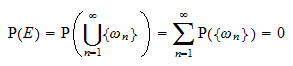sincefor every.

Therefore,is a zero-probability event.

This might seem surprising: in this probability model there are zero-probability events comprising infinitely many sample points!

It can also easily be proved that the event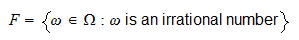is an almost sure event.

In fact,and by applying the formula for the probability of a complement, we get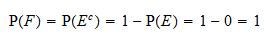## Solved exercises

Below you can find some exercises with explained solutions.

### Exercise 1

Letandbe two events.

Letbe a zero-probability event andCompute.

Solutionis a zero-probability event, which means thatFurthermore, using the formula for the probability of a complement, we obtainSince, by monotonicity we obtainSinceand probabilities cannot be greater than, this implies### Exercise 2

Letandbe two events.

Letbe a zero-probability event andCompute.

Solutionis a zero-probability event, which means thatFurthermore, using the formula for the probability of a complement, we obtainIt is also true that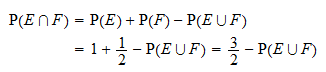Since, by monotonicity, we obtainSinceand probabilities cannot be greater than, this impliesThus, putting pieces together, we get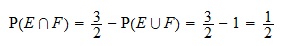## References

Williams, D. (1991) Probability with martingales, Cambridge University Press.